# MeshTorus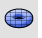Mesh

Polygon Mesh Primitives >

Torus

The MeshTorus command draws a polygon mesh torus.Base circle options

Pick a point or enter a radius to decide the size of the base circle.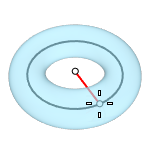##### Vertical

Draws the base circle vertical to the construction plane.##### 2Point

Pick two opposite points on the base circle.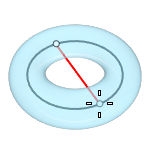##### 3Point

Draws the base circle through three points.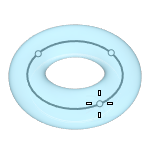##### Tangent

Draws the base circle tangent to one, two, or three curves.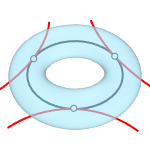##### AroundCurve

Draws the base circle perpendicular to the picked point on a curve. The center line of the torus will be tangent to the curve.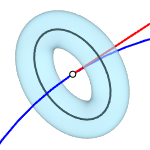##### FitPoints

Draws the base circle by fitting to selected points, control points, or mesh vertices.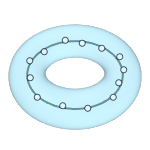### VerticalFaces

The number of faces along the second circle.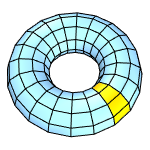### AroundFaces

The number of faces along the base circle.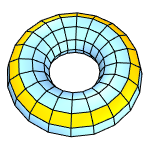Second circle options

Pick a point or enter a radius to decide the size of the second circle.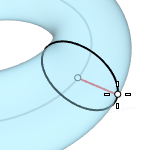##### Diameter

Pick a point or enter a diameter to decide the size of the second circle.##### FixInnerDimension

The first radius chosen sets the inner dimension of the torus and the second radius is constrained to be outside of the first radius.#####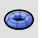SubDTorusCreates a subdivision torus object.

#####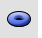Torus

Draw a NURBS torus (donut shape).

Rhinoceros 7 © 2010-2022 Robert McNeel & Associates. 16-Aug-2022